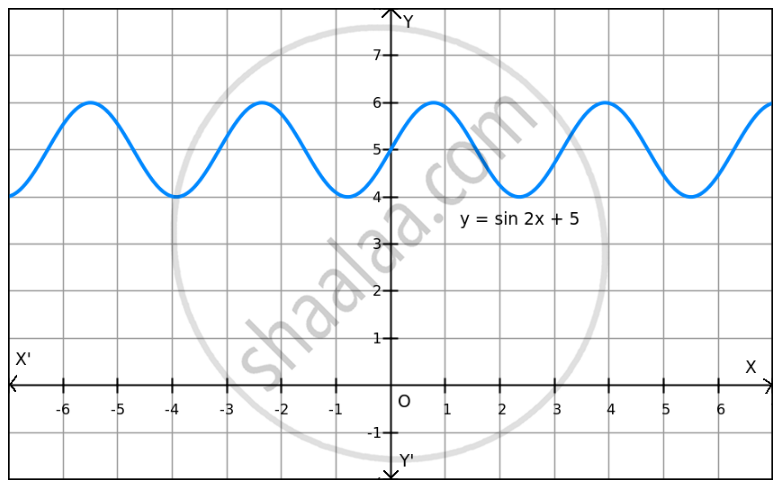PUC Karnataka Science Class 12Department of Pre-University Education, Karnataka
Share

F(X)=Sin 2x+5 on R . - PUC Karnataka Science Class 12 - Mathematics

Question

f(x)=sin 2x+5 on R .

Solution

Given: f(x) = sin 2x + 5

We know that − 1 ≤ sin 2x ≤ 1.

⇒ − 1 + 5 ≤ sin 2x + 5 ≤ 1 + 5

⇒ 4 ≤ sin 2x + 5 ≤ 6
⇒ 4 ≤ f(x) ≤ 6Hence, the maximum and minimum values of f are 6 and 4, respectively.

Is there an error in this question or solution?

Video TutorialsVIEW ALL 

Solution F(X)=Sin 2x+5 on R . Concept: Graph of Maxima and Minima.
S